Elliott Sound Products AN-008

How to Use Zener Diodes

Rod Elliott (ESP)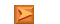App. Notes IndexMain Index

Zener diodes are very common for basic voltage regulation tasks. They are used as discrete components, and also within ICs that require a reference voltage. Zener diodes (also sometimes called voltage reference diodes) act like a normal silicon diode in the forward direction, but are designed to break down at a specific voltage when subjected to a reverse voltage.

All diodes will do this, but usually at voltages that are unpredictable and much too high for normal voltage regulation tasks. There are two different effects that are used in Zener diodes ...

• Impact ionisation (also called avalanche breakdown) - positive temperature coefficient
• Zener breakdown - negative temperature coefficient

Below around 5.5 Volts, the zener effect is predominant, with avalanche breakdown the primary effect at voltages of 8V or more. While I have no intention to go into specific details, there is a great deal of information on the Net (See References) for those who want to know more. Because the two effects have opposite thermal characteristics, zener diodes at close to 6V usually have very stable performance with respect to temperature because the positive and negative temperature coefficients cancel.

Very high thermal stability can be obtained by using a zener in series with a normal diode. There are no hard and fast rules here, and it normally requires device selection to get the combination to be as stable as possible. A zener of around 7-8V can be selected to work with a diode to cancel the temperature drift. Needless to say, the diode and zener junctions need to be in intimate thermal contact, or temperature cancellation will not be a success.

The zener diode is a unique semiconductor device, and it fulfils many different needs unlike any other component. A similar device (which is in fact a specialised zener itself) is the TVS (transient voltage suppressor) diode. There are several alternatives to TVS diodes though, unlike zeners. Precision voltage reference ICs may be thought of as being similar to zeners, but they aren't - they are ICs that use a bandgap reference (typically around 1.25V). These are ICs, containing many internal parts. A zener diode is a single part, with a single P-N junction.

Using Zener Diodes
For reasons that I don't understand, there is almost no information on the Net on exactly how to use a zener diode. Contrary to what one might expect, there are limitations on the correct usage, and if these are not observed, the performance will be much worse than expected. Figure 1 shows the standard characteristics of a zener, but as with almost all such diagrams omits important information.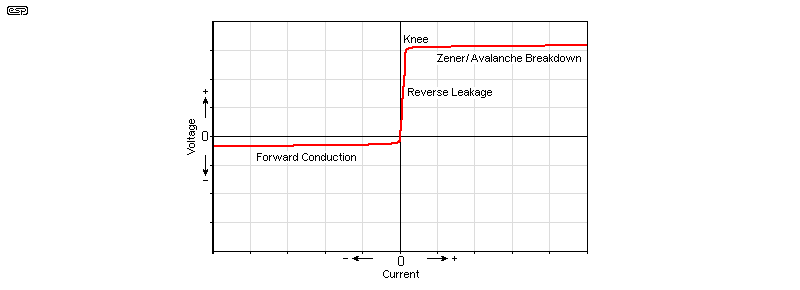Figure 1 - Zener Diode Conduction

So, what's missing? The important part that is easily missed is that the slope of the breakdown section is not a straight line. Zeners have what is called 'dynamic resistance' (or impedance), and this is something that should be considered when designing a circuit using a zener diode.

The actual voltage where breakdown starts is called the knee of the curve, and at this region the voltage is quite unstable. It varies quite dramatically with current, so it is important that the zener is operated above the knee, where the slope is most linear.

Some data sheets will give the figure for dynamic resistance, and this is usually specified at around 0.25 of the maximum rated current. Dynamic resistance can be as low as a couple of ohms at that current, with zener voltages around 5 - 6V giving the best result. Note that this coincides with the best thermal performance as well.

This is all well and good, but what is dynamic resistance? It is simply the 'apparent' resistance that can be measured by changing the current. This is best explained with an example. Let's assume that the dynamic resistance is quoted as 10 ohms for a particular zener diode. If we vary the current by 10mA, the voltage across the zener will change by ...

V = R × I   = 10Ω * 10mA = 0.1V (or 100mV)

So the voltage across the zener will change by 100mV for a 10mA change in current. While that may not seem like much with a 15V zener for example, it still represents a significant error. For this reason, it is common to feed zeners in regulator circuits from a constant current source, or via a resistor from the regulated output. This minimises the current variation and improves regulation.

Manufacturers' data sheets will often specify the dynamic resistance at both the knee and at a specified current. It is worth noting that while the dynamic resistance of a zener may be as low as 2-15 ohms at 25% of maximum current (depending on voltage and power ratings), it can be well over 500 ohms at the knee, just as the zener starts to break down. The actual figures vary with breakdown voltage, with high voltage zeners having very much higher dynamic resistance (at all parts of the breakdown curve) than low voltage units. Likewise, higher power parts will have a lower dynamic resistance than low power versions (but require more current to reach a stable operating point).

Finally, it is useful to look at how to determine the maximum current for a zener, and establish a rule of thumb for optimising the current for best performance. Zener data sheets usually give the maximum current for various voltages, but it can be worked out very easily if you don't have the datasheet to hand ...

I = P / V     where I = current, P = zener power rating, and V = zener voltage rating.

For example, a 27V 2W zener can carry a maximum continuous current of ...

I = 2 / 27 = 0.074A = 74mA (at 25°C)

As noted in the 'transistor assisted zener' app note (AN-007), for optimum zener operation, it is best to keep the current to a maximum of 0.7 of the rated current, so a 27V/2W zener should not be run at more than about 47mA. The ideal is between 20-30% of the maximum , as this minimises wasted energy, keeps the zener at a reasonable temperature, and ensures that the zener is operating within the most linear part of the curve. If you look at the zener data table below, you will see that the test current is typically between 25% and 36% of the maximum continuous current. The wise reader will figure out that this range has been chosen to show the diode in the best possible light, and is therefore the recommended operating current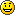.

While none of this is complex, it does show that there is a bit more to the (not so) humble zener diode than beginners (and many professionals as well) tend to realise. Only by understanding the component you are using can you get the best performance from it. This does not only apply to zeners of course - most (so called) simple components have characteristics of which many people are unaware.

Remember that a zener is much the same as a normal diode, except that it has a defined reverse breakdown voltage that is far lower than any standard rectifier diode. Zeners are always connected with reverse polarity compared to a rectifier diode, so the cathode (the terminal with the band on the case) connects to the most positive point in the circuit.

Zener Clamps

Often, it is necessary to apply a clamp to prevent an AC voltage from exceeding a specified value. Figure 2 shows the two ways you may attempt this. The first is obviously wrong - while it will work as a clamp, the peak output voltage (across the zeners) will only be 0.65V. Zeners act like normal diodes with reverse polarity applied, so the first figure is identical to a pair of conventional diodes.Figure 2 - Zener Diode AC Clamp

In the first case, both zener diodes will conduct as conventional diodes, because the zener voltage can never be reached. In the second case, the actual clamped voltage will be 0.65V higher than the zener voltage because of the series diode. 12V zeners will therefore clamp at around 12.65V - R1 is designed to limit the current to a safe value for the zeners, as described above.

The important thing to remember is that zener diodes are identical to standard diodes below their zener voltage - in fact, conventional diodes can be used as zeners. The actual breakdown voltage is usually much higher than is normally useful, and each diode (even from the same manufacturing run) will have a different breakdown voltage that is normally far too high to be useful.

Zener Diode Data

The data below is fairly typical of 1W zeners in general, and shows the zener voltage and one of the most important values of all - the dynamic resistance. This is useful because it tells you how well the zener will regulate, and (with a bit of calculation) how much ripple you'll get when the zener is supplied from a typical power supply. An example of the calculation is shown further below.

If if you wanted to measure the dynamic resistance for yourself, it's quite easy to do. First, use a current of about 20% of the rated maximum from a regulated power supply via a suitable resistor. Measure and note down the voltage across the zener diode. Now, increase the current by (say) 10mA for zeners less than 33V. You'll need to use a smaller current increase for higher voltage types. Measure the zener voltage again, and note the exact current increase.

For example, you might measure the following ...

Zener voltage = 11.97 V at 20 mA
Zener voltage = 12.06 V at 30 mA
ΔV = 90 mV, ΔI = 10 mA
R = ΔV / ΔI = 0.09 / 0.01 = 9 ohms

This process can be used with any zener. You just need to adjust the current to suit, ensuring that the initial and final test currents are within the linear section of the zener's characteristics. The accuracy depends on the accuracy of your test equipment, and it's important to ensure the zener temperature remains stable during the test or you'll get the wrong answer due to the zener's thermal coefficient. If at all possible, the tests should be of very short duration using pulses, but this is very difficult without specialised equipment.

The following data is a useful quick reference for standard 1W zeners. The basic information is from the Semtech Electronics data sheet for the 1N47xx series of zeners. Note that an 'A' suffix (e.g. 1N4747A) means the tolerance is 5%, and standard tolerance is usually 10%. Zener voltage is measured under thermal equilibrium and DC test conditions, at the test current shown (Izt).

Notice that the 6.2V zener (1N4735) has the lowest dynamic resistance of all those shown, and will generally also show close to zero temperature coefficient. This means that it is one of the best values to use where a fairly stable voltage reference is needed. Because it's such a useful value, it has been highlighted in the table. If you need a really stable voltage reference then don't use a zener diode, but use a dedicated precision voltage reference IC instead.

TypeVZ (Nom) IZt mARZt Ω RZ Ω at ...Knee
Current
(mA)
Leakage
µA
Leakage
Voltage
Peak
Current (mA)
Cont.
Current (mA)
1N47283.37610400115011375275
1N47293.66910400110011260252
1N47303.9649.0400110011190234
1N47314.3589.040015011070217
1N47324.7538.05001101970193
1N47335.1497.05501101890178
1N47345.6455.06001102810162
1N47356.2412.07001103730146
1N47366.8373.57001104660133
1N47377.5344.07000.5105605121
1N47388.2314.57000.5106550110
1N47399.1285.07000.5107500100
1N474010257.07000.25107.645491
1N474111238.07000.2558.441483
1N474212219.0 7000.2559.138076
1N47431319107000.2559.934469
1N47441517147000.25511.430461
1N47451615.5167000.25512.228557
1N47461814207500.25513.725050
1N47472012.5227500.25515.222545
1N47482211.5237500.25516.720541
1N47492410.5257500.25518.219038
1N4750279.5357500.25520.617034
1N4751308.54010000.25522.815030
1N4752337.54510000.25525.113527
1N4753367.05010000.25527.412525
1N4754396.56010000.25529.711523
1N4755436.07015000.25532.711022
1N4756475.58015000.25535.89519
1N4757515.09515000.25538.89018
1N4758564.511020000.25542.68016
1N4759624.012520000.25547.17014
1N4760683.715020000.25551.76513
1N4761753.317520000.25556.06012
1N4762823.020030000.25562.25511
1N4763912.825030000.25569.25010
1N47641002.535030000.25576.0459
Table 1 - Zener Characteristics, 1N4728-1N4764
1. IZt = zener test current
2. RZt = dynamic resistance at the stated test current
3. RZ = dynamic resistance at the current shown in the next column (Knee Current (mA))
4. Leakage current = current through the zener below the knee of the zener conduction curve, at the voltage shown in the next column (Leakage Voltage)
5. Peak current = maximum non-repetitive short term current (typically < 1ms)
6. Continuous current = maximum continuous current, assuming that the leads at 10mm from the body are at 25°C (highly unlikely in practice)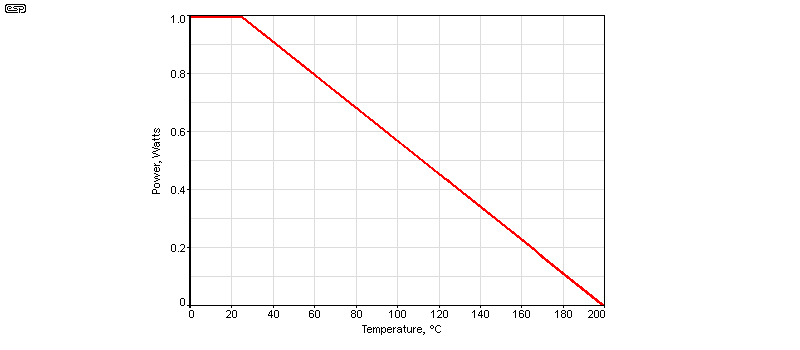Figure 3 - Zener Diode Temperature Derating

Like all semiconductors, zeners must be derated if their temperature is above 25°C. This is always the case in normal use, and if the guidelines above are used then you usually won't need to be concerned. The above graph shows the typical derating curve for zener diodes, and this must be observed for reliability. Like any other semiconductor, if a zener is too hot to touch, it's hotter than it should be. Reduce the current, or use the boosted zener arrangement described in AN-007.

Zener diodes can be used in series, either to increase power handling or to obtain a voltage not otherwise available. Do not use zeners in parallel, as they will not share the current equally (remember that most are 10% tolerance). The zener with the lower voltage will 'hog' the current, overheat and fail. When used in series, try to keep the individual zener voltages close to the same, as this ensures that the optimum current through each is within the optimum range. For example, using a 27V zener in series with a 5.1V zener would be a bad idea because the optimum current through both cannot be achieved easily.

Putting Zeners To Use

Using zener diodes as regulators is easy enough, but there are some things that you need to know before you wire everything up. A typical circuit is shown below for reference, and is not intended to be anything in particular - it's simply an example. Note that if you need a dual supply (e.g. ±15V), then the circuit is simply duplicated for the negative supply, reversing the polarity of the zener and C1 as required. We will use a 1W zener, in this case a 1N4744, a 15V diode. The maximum current we'd want to use is about half the calculated maximum (no more than 33mA). The minimum acceptable current is around 10% (close enough to 7mA).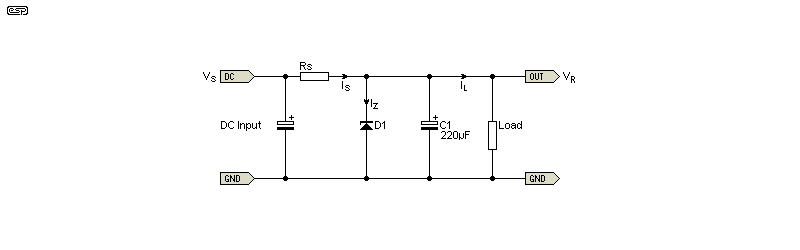Figure 4 - Typical Zener Regulator Circuit

Firstly, you need to know the following details about your intended circuit ...

1. Source voltage - from a power amplifier supply for example (including any ripple voltage)
2. Maximum and minimum values of the source voltage - it will vary depending on mains voltage, load current and ripple
3. Desired regulated voltage - preferably using a standard value zener. We'll use 15V
4. Load current - the expected current drain of the circuitry powered from the zener regulated supply

When you have this information, you can determine the series resistance needed for the zener and load. The resistor needs to pass enough current to ensure that the zener is within its linear region, but well below the maximum to reduce power dissipation. If the source voltage varies over a wide range, it may not be possible to use a simple zener regulator successfully.

Let's assume that the source voltage comes from a 35V power supply used for a power amplifier. The maximum voltage might be as high as 38V, falling to 30V when the power amp is driven to full power at low mains voltage. Meanwhile, the preamp that needs a regulated supply uses a pair of opamps, and draws 10mA. You want to use a 15V supply for the opamps. This is all the required info, so we can do the calculations. Vs is source voltage, Is is source current, Iz is zener current, IL is load current and Rs is source resistance.

Iz (max) = 30mA (worst case, no load on main supply and maximum mains voltage)
IL = 10mA (current drawn by opamps)
Is (max) = 40mA (again, worst case total current from source)

From this we can work out the resistance Rs. The voltage across Rs is 23V when the source voltage is at its maximum, so Rs needs to be ...

Rs = Vs / I = 23 / 40m = 575 ohms

When the source voltage is at its minimum, there will be only 15V across Rs, so we need to check that there will still be enough zener current ...

Is = V / R = 15 / 575 ohms = 26mA
Iz = Is - IL = 26mA - 10mA = 16mA

When we take away the load current (10mA for the opamps), we still have a zener current of 16mA available, so the regulation will be quite acceptable, and the zener diode won't be stressed. 575 ohms is not a standard value, so we'd use a 560 ohm resistor instead. There's no need to re-calculate everything because the change is small and we were careful to ensure that the design was conservative to start with. The next step is to work out the worst case power dissipated in the source resistor Rs ...

Is = 23V / 560 ohms = 41mA P = Is² × R = 41mA² * 560 ohms = 941mW

In this case, it would be unwise to use less than a 2W resistor, but a 5W wirewound type would be better. In the same way as the resistor power was calculated, it's also a good idea to double check the zener's worst case dissipation. It may be possible to disconnect the opamps, in which case the zener will have to absorb the full 41mA, so dissipation will be 615mW. That's higher than the target set at the beginning of this exercise, but it's within the zener's 1W rating and will never be a long-term issue. Normal worst case dissipation is only 465mW when the opamps are connected, and that's quite acceptable.

Figure 4 shows a 220µF capacitor in parallel with the zener. This does not make any appreciable difference to the output noise, because the impedance (aka dynamic resistance) of the zener is so low. We used an example of a 15V zener, so we expect it's impedance to be about 14 ohms (from the table). To be useful at reducing noise, C1 would need to be at least 1,000uF, but in most cases much lower values are used (typically 100-220uF). The purpose is to supply instantaneous (pulse) current that may be demanded by the circuit, or in the case of opamps, to ensure that the supply impedance remains low up to at least 2MHz or so.

Because zener diodes have a dynamic resistance, there will be some ripple at the output. It's possible to calculate it based on the input ripple, change of source current and the zener's dynamic resistance. Let's assume that there is 2V P-P ripple on the source voltage. That means the current through Rs will vary by 3.57mA ( I = V / R ). The zener has a dynamic resistance of 14 ohms, so the voltage change across the zener must be ...

V = R × I = 14 × 3.57m = 50mV peak-peak (less than 20mV RMS)

Provided the active circuitry has a good power supply rejection ratio (PSRR), 20mV of ripple at 100Hz (or 120Hz) will not be a problem. If that can't be tolerated for some reason, then it's cheaper to use a 3-terminal regulator than to use any of the established methods for ripple reduction. The most common of these is to use two resistors in place of Rs, and place a high value cap (not less than 470µF) from the junction of the resistors to ground. Doing this will reduce the ripple to well below 1mV, depending on the size of the extra capacitor.

Maximising Stability (Voltage Reference)

The standard resistor zener feed is subject to wide variations of current and power dissipation as the input voltage varies. A simple feedback circuit can help to maintain a very stable current through the zener, and therefore provide a more stable reference voltage. As discussed earlier, a 6.2V zener diode has a very low thermal coefficient of voltage, and if we can ensure it gets a stable current, this further improves the voltage regulation. Feeding a zener with a current source is standard practice in IC fabrication, and it's easy enough to do in discrete designs as well.

The arrangement shown below is not intended for use as a power supply, but to provide a fixed reference voltage for other circuitry that may require a stable voltage for comparators (for example). The circuit can't compete with a dedicated precision voltage reference, but it will be surprisingly good for many general purpose applications. The current mirror (Q2b and Q3b) is fed from a current source (Q1b) which takes its reference from the zener, so there is a closed loop and the current variation through the zener itself can be very small. With the values shown, the zener current is only 2.5mA, which seems to defy the guidelines given earlier. However, increasing the zener current doesn't help a great deal, but it increases dissipation in the transistors. For example, if R1b is reduced to 1k, the zener current is increased to 5.4mA, dissipation in Q1b and Q3b is doubled, but the regulation is only improved marginally.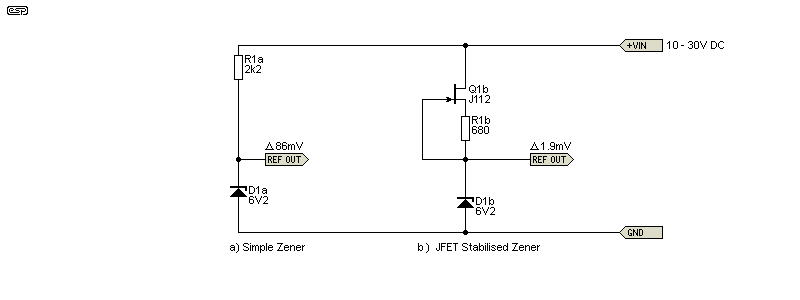Figure 5 - 'Conventional' Vs. Precision Zener Regulator Circuit

Compare (a) and (b) in the Figure 5 circuits, and it's immediately apparent that the voltage from the stabilised version (b) should be very stable indeed, even with a wide variation of input voltage. Simulated over a voltage range from 10V up to 30V, there is less than a 3mV voltage change across the zener, and it follows that zener current and zener power dissipation barely change over the entire voltage range. This also means that ripple rejection is extremely high, so with the addition of three cheap transistors and four resistors, we can get close to a real precision voltage reference circuit. R4b is needed so the circuit can start when voltage is applied, but unfortunately it does adversely affect the performance. A higher resistance reduces the effects, but may cause unreliable start-up.

The standard zener regulator (a) will show a typical voltage change of around 110mV from an input voltage of 10-30V, with zener current changing from 1.7mA to over 15mA. This is significantly worse than the stabilised version, but it may not represent a problem at all if the input voltage is fairly stable. In reality, it's unlikely that you will ever need to use the more complex stabilised zener, and it's included here purely in the interests of completeness.

References
1   Reverse Biased / Breakdown - Discussing the phenomenon when the diode is reverse biased/breakdown. Bill Wilson
2   RadioElectronics.com - Summary of the zener diode
3   Data Sheet Archive - BZX2C16V Micro Commercial Components 2 Watt Zener Diode 3.6 to 200 Volts.
4   Zener Diode Theory - OnSemi Handbook HBD854/D (No longer available from OnSemi.)App. Notes IndexMain Index

 Copyright Notice.This article, including but not limited to all text and diagrams, is the intellectual property of Rod Elliott, and is Copyright © 2004. Reproduction or re-publication by any means whatsoever, whether electronic, mechanical or electro-mechanical, is strictly prohibited under International Copyright laws. The author (Rod Elliott) grants the reader the right to use this information for personal use only, and further allows that one (1) copy may be made for reference while constructing the project. Commercial use is prohibited without express written authorisation from Rod Elliott.
Page Created and Copyright © Rod Elliott 30 Jun 2005./ Jul 2015 - Updated info, added Figure 4.